1. What is the value of (222+22 )  ÷ 2 ?

A:
B:
C:
D:
E:

2. A train carriage has 80 seats. On my journey I noticed that all the seats in my carriage were taken and 7 people were standing.

At Banbury, 9 people left the carriage, 28 people entered it and all the seats were taken.

How many people now had no seat?

A:
B:
C:
D:
E:

3. The diagram shows an equilateral triangle, a square, and one diagonal of the square.

What is the value of x ?

A:
B:
C:
D:
E:

4. The perimeter of the regular decagon P is 8 times the perimeter of the regular octagon Q.

Each edge of the regular octagon Q is 10 cm long.

How long is each edge of the regular decagon P?

5. My train left Southampton at 06:15 and arrived in Birmingham at 08:48 later that morning.

How many minutes did the journey take?

A:
B:
C:
D:
E:

6. The diagram shows a partially completed magic square, in which all rows, all columns and both main diagonals have the same total.

What is the value of x + y?

A:
B:
C:
D:
E:

7. How many integers are greater than 20 + 18 and also less than 20 × 18?

A:
B:
C:
D:
E:

8. Gill scored a goal half way through the second quarter of a ‘teachers versus pupils’ netball match.

At that point, what fraction of the whole match remained to be played?

A: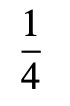B: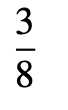C: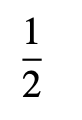D: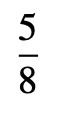E: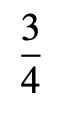9. The approximate cost of restoring the Flying Scotsman was £4 million. This was about 500 times the cost of building the steam engine in 1923.

Roughly what did the engine cost to build?

10.

Which of the fractions is not used?

A:B: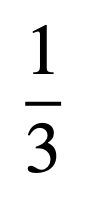C: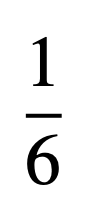D: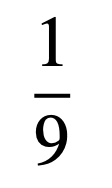E: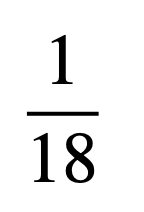11. How many digits are there in the correct answer to the calculation 123 123 123 123 ÷ 123 ?

A:B:C:D:E:12. The diagram shows a quadrilateral PQRS in which PQ and QR have the same length. Also PR bisects ∠SPQ, the ratio of ∠SPR to ∠PRS is 2 : 3 and ∠PSR = 110°.

How large is angle PQR?

A:B:C:D:E:13. The diagram shows a shape made from four 3 cm × 3 cm × 3 cm wooden cubes joined by their edges.

What, in cm², is the surface area of the shape?

A:B:C:D:E:14. Billy has three times as many llamas as lambs. Milly has twice as many lambs as llamas.

They have 17 animals in total.

How many of the animals are llamas?

A:B:C:D:E:15. Beatrix places copies of the L-shape shown on a 4 × 4 board so that each L-shape covers exactly three cells of the board.

She is allowed to turn around or turn over an L-shape.

What is the largest number of L-shapes she can place on the board without overlaps?

A:B:C:D:E:Back to the top